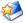# [原创]载人登月是一项严肃的国家工程

[size=10.5]载人登月是一项严肃的国家工程[size=10.5]
[size=10.5]
[size=10.5]第一节，目前版本的长征[size=10.5]5[size=10.5]号火箭实际上己经等于推倒重来。
[size=10.5]坦率而言，目前版本的长征[size=10.5]5[size=10.5]号火箭实际上己经等于彻底推倒重来了。并联[size=10.5]7[size=10.5]台[size=10.5]FY100K[size=10.5]发动机研制的全新[size=10.5]5[size=10.5]米直径主芯火箭只要研制成功。目前版本的长征[size=10.5]5[size=10.5]号火箭也注定要同时走到了历史的尽头。
[size=10.5]明确无误地说，目前版本的长征[size=10.5]5[size=10.5]号火箭己经失去了政府高层的信任，因此在当前的环境下再讨论目前版本的长征九号火箭的研制方案已经完全是在浪费时间。现在一院最大的责任与最大的压力就是如何确保目前版本的长征[size=10.5]5[size=10.5]号火箭在发射嫦娥五号、嫦娥六号探测月飞船与天宫空间站的核心舱、实验舱那几发火箭不再出漏子就万事大吉。[size=10.5]
[size=10.5]
[size=10.5]而目前版本的长征五号火箭己经成为烫手的山芋，一院作为局内人自然要比局外人更为清楚明白。现在一院的赦远平院长都直说“压力巨大”。[size=10.5]
[size=10.5]
[size=10.5]长征五号火箭的这个坑挖的太深、技术方案太过激进了。最后搞得所有与长征五号火箭研制相关的人员现在都如履薄冰、压力巨大，乃至赌上自己的前途命运。这也实在太过失败了。不就是一款[size=10.5]GTO[size=10.5]运力不过区区[size=10.5]14[size=10.5]吨的火箭吗？！为何却要同时使用上[size=10.5]3[size=10.5]款完全不同的发动机？！这完全是要逼死设计师的技术方案（而目前版本的长征九号火箭研制方案则又完全重复了长征五号火箭的所有致命缺点）。[size=10.5]
[size=10.5]
[size=10.5]对于目前版本的长征[size=10.5]5[size=10.5]号火箭，政府高层己经明显失去了信心，长征七号由于在内陆发射场还能使用，发展不受影响。高凉陈君早前还认为会改为使用[size=10.5]4[size=10.5]台[size=10.5]YF77[size=10.5]发动机并联研制改进型长征五号，将目前版本的长征[size=10.5]5[size=10.5]号氢氧主芯级火箭“撑”下去。但现在高层己经明显否决了这一方案。最后转了个大弯，还是直接研制全煤油[size=10.5]5[size=10.5]米直径主芯级火箭就了事。
[size=10.5]
[size=10.5]而长征五号火箭的研制“转”了一大圈弯路，浪费了一百多亿人民币与[size=10.5]10[size=10.5]年时间，最后还是回到了全煤油发动机的[size=10.5]5[size=10.5]米直径主芯级的初始设计技术路径上。这能够怪谁？！其中的教训还不足够深刻？！[size=10.5]
[size=10.5]
[size=10.5]目前版本的长五火箭己经让各方失去了继续等待的耐心。想想下也是，目前长五构型的[size=10.5]YF77[size=10.5]氢氧发动机主芯级与[size=10.5]YF100[size=10.5]煤油发动机助推器都是完全没有冗余的产物，起飞级的[size=10.5]10[size=10.5]台发动机中只要有一台出现故障，发射就只能够完败。这种娘胎里就带来的缺点也不是小修小补能够解决的。最后就只有快刀斩乱麻，推倒重来就了事。[size=10.5]
[size=10.5]
[size=10.5]细细分析也是如此，改为使用[size=10.5]4[size=10.5]台[size=10.5]YF77[size=10.5]氢氧发动机并联升级目前版本的长五主芯级，工作量也一点不比目前使用[size=10.5]7[size=10.5]台[size=10.5]YF100K[size=10.5]煤油发动机并联研制[size=10.5]5[size=10.5]米直径主芯级要小，但运力拓展区间与可靠性冗余却差距极大，好处也仅仅是保住了[size=10.5]YF77[size=10.5]氢氧发动机的持续使用机会而已。但高层现在明显对[size=10.5]YF77[size=10.5]氢氧发动机失去了耐心，而且猎鹰[size=10.5]9[size=10.5]火箭崛起后一次性火箭起飞级使用氢氧发动机的经济性更是完败。
[size=10.5]
[size=10.5]米申背了原苏联[size=10.5]N1[size=10.5]火箭的锅，[size=10.5]NASA[size=10.5]局长格里芬背了美国战神[size=10.5]1[size=10.5]、战神五系列火箭的锅，而李东则背了中国长征五号火箭的锅。这种教训难道还不够深刻吗？！一种火箭搞得太过复杂，技术方案太过激进，实际上就是在为自己挖了一个深深的大坑，最后再将自己整个埋入去而已。
[size=10.5]还能什么办？！现在只能死鸡撑硬脚，小修小补下，再死撑到并联[size=10.5]7[size=10.5]台[size=10.5]YF100K[size=10.5]煤油发动机的新火箭研制成功为止。[size=10.5]
[size=10.5]
[size=10.5]日本的[size=10.5]H2[size=10.5]到[size=10.5]H2A[size=10.5]火箭升级改进当年也是这么走过来的。这也是没有办法的事。并联[size=10.5]2[size=10.5]台[size=10.5]YF77[size=10.5]氢氧发动机研制的主芯级的价格什么也要比并联[size=10.5]7[size=10.5]台[size=10.5]FY100K[size=10.5]煤油发动机的[size=10.5]5[size=10.5]米主芯级的价格还要贵。因此一旦并联[size=10.5]7[size=10.5]台[size=10.5]YF100K[size=10.5]煤油发动机的新火箭研制成功，目前版本的长征五号火箭那能同其比拼“性价比”？！也只能够迅速退役就了事。[size=10.5]

打赏
收藏文本
0
0
2018/11/4 1:10:19

## 网友回复

左箭头-小图标

因此，高凉陈君估计中国的YF77氢氧发动机的市场价格至少在1000万美元一台，而YF100煤油发动机的市场价格则处于300万美元一台的区间。
更为重要的是，参考NK15NK33、梅林1RD107RD108煤油发动机的生产工艺技术，全球100多吨推力区间的煤油发动机要大规模扩大产能似乎并不存在“瓶颈制约”。只要有充足的发射需求与采购资金，象俄罗斯要将NK33RD107RD108发动机的年产量提升到美国梅林1发动机的400台的规模水平也是易如反掌的事情。
而且现实中航天六院的YF100煤油发动机的产量也要远远超过YF77氢氧发动机。
因此，中国的YF100K煤油发动机未来也能够象YF20肼燃料发动机与美国的梅林1煤油发动机那样，通过扩大生产规模来大幅度“摊薄”每一台发动机的生产成本。
YF77氢氧发动机要想大幅度扩大生产规模来“摊薄产品成本”，难度就要大得多。至少那个采用管道壁冷却技术的再生冷却喷管就没有那么容易扩大产量规模。
美国洛克达因公司作为全球氢氧发动机领域的生产霸主，在研制RS68巨型氢氧发动机时也只能够改为走烧蚀喷管的技术路径来解决，（也因此而大大降低了RS68氢氧发动机的持续工作时间能力）。
第三节，研制载人登月火箭是一项严肃的政治工程。
[size=10.5]高凉陈君对发动机生产技术不堪理解，只是直观感觉《大国工匠》中[size=10.5]YF77[size=10.5]氢氧发动机的喷管生产非常复杂麻烦，效率很低。[size=10.5]
[size=10.5]
[size=10.5]还有液氢的超底温物理特性决定了氢氧液体火箭不好伺候，其的发射场整备也非常麻烦与成本昂贵。这也是[size=10.5]ULA[size=10.5]宁可停掉现成的德尔塔[size=10.5]4[size=10.5]火箭再转而研制火神火箭的最重要原因。[size=10.5]
[size=10.5]
[size=10.5]长征五号火箭早期立项论证的文件就是典型的屁股决定脑袋，即为了上马[size=10.5]YF77[size=10.5]氢氧发动机不惜一切代价与不惜一切手段，同今天的长征九号火箭的上马研制支持者一模一样。当年论证长征[size=10.5]5[size=10.5]号火箭时院士们还说一级半方案能够大大提高火箭的工作可靠性，而现实中[size=10.5]YF77[size=10.5]氢氧发动机在长五遥[size=10.5]2[size=10.5]任务中却在[size=10.5]346[size=10.5]秒就出故障完蛋了。一级半设计方案才是现在“坑死”长征[size=10.5]5[size=10.5]号火箭的最重要根源。[size=10.5]
[size=10.5]
[size=10.5]如果当年研制长征五号火箭时还是走长二捆火箭的成熟技术路径，即使用[size=10.5]4[size=10.5]台或者[size=10.5]5[size=10.5]台[size=10.5]YF100[size=10.5]煤油发动机并联研制[size=10.5]5[size=10.5]米直径的主芯级，再捆绑[size=10.5]4[size=10.5]枚[size=10.5]2[size=10.5]点[size=10.5]25[size=10.5]米直径的[size=10.5]YF100[size=10.5]煤油助推器。一款[size=10.5]LEO[size=10.5]运力[size=10.5]20[size=10.5]多吨，[size=10.5]GTO[size=10.5]运力[size=10.5]10[size=10.5]多吨的重型火箭至少能够早[size=10.5]7[size=10.5]、[size=10.5]8[size=10.5]年时间就研制成功，也就没有今天的那么多的麻烦事了。因此李东、王钰们才是真正的背锅侠，倒是余梦伦、龙乐豪等老院士们什么事也没有。好在今天国家处于上升期，几百亿元花就花了，高层也没说什么，大家“罚酒三杯”而已。[size=10.5]
[size=10.5]
[size=10.5]所以国家强大才是硬道理，才能够让科学家们有足够多的试错机会。那怕项目失败了国家也不会伤筋动骨。但从长征五号到长征九号火箭的方案却一错再错，龙乐豪等老院士们也末免太过轻看高层的智慧了，也末免太过以为高层“不懂火箭技术”了。现在[size=10.5]921[size=10.5]办公室就直接决定末来中国载人登月火箭的构型方案，不再任由龙乐豪们天马行空，随心所欲。这是一个重要的政治信号，相关老院士们必须学会自重了。[size=10.5]
[size=10.5]
[size=10.5]现在问题的关键是[size=10.5]ULA[size=10.5]公司也决定抛弃现成的德尔塔[size=10.5]4[size=10.5]氢氧火箭，这表明氢氧火箭一定还存在其他的重大经济成本缺憾。目前[size=10.5]ULA[size=10.5]公司对宇宙神[size=10.5]5[size=10.5]火箭的喜爱是明确无误的，[size=10.5]ULA[size=10.5]捞到的几单商业卫星发射合同也全部都是由宇宙神五来执行。如果宇宙神[size=10.5]5[size=10.5]火箭不是使用产自俄罗斯的[size=10.5]RD180[size=10.5]发动机的话。高凉陈君认为宇宙神[size=10.5]5[size=10.5]至少还会持续使用上三、四十年时间。[size=10.5]
[size=10.5]
[size=10.5]是的，所以现在高层对长征五号火箭的推倒重来也没有责怪主持论证长五项目的科学家的意思，权当是交了一笔高达几百亿元的“昂贵学费”而已。大家“罚酒三杯”就[size=10.5]OK[size=10.5]了事。但今天[size=10.5]921[size=10.5]办公室代表政府高层指定中国载人火箭的具体构型方案之后，龙乐豪等老院士们就必须深刻理解上面的苦心与难处，不要再自讨没趣与不爽快了。希望一院看到本文的人士转告老院士们一声，载人登月不同于研制长征五号火箭。不要再瞎起哄掺和了，好好过好体面晚年生活不好吗？！[size=10.5]
[size=10.5]
[size=10.5]洛克达因公司无论生产什么都不会便宜，这才真正是美国的体制问题，但俄罗斯与蓝色起源公司能够生产出便宜的烃燃料发动机，这也真正表明烃类燃料发动机真的是能够便宜生产出来的。而随着中国的长征五号与美国的德尔塔[size=10.5]4[size=10.5]火箭逐渐退出现役，未来只有阿里安[size=10.5]6[size=10.5]与[size=10.5]H3[size=10.5]坚守氢氧主芯级火箭了。考虑到阿里安下一代火箭也是走甲烷发动机的路线，最后也只有日本[size=10.5]H3[size=10.5]“死撑”了。而日本又是一个非常另类的国家，不能以平常眼光来评论之。[size=10.5]
[size=10.5]
[size=10.5]龙乐豪老院士所推出的重型火箭方案都不知道有多少款了（至少有十几种之多）。高凉陈君都不知道龙院士为何就那么轻易就签署上他的大名，他至少也是院士，历史知名人物，理应严肃下才对的。当然，由于目前版本的长征[size=10.5]5[size=10.5]号火箭己经推倒重来，以后再不严肃点，后果就会非常难看了，而且恐怕还会有其他风险隐患。[size=10.5]
[size=10.5]
[size=10.5]一直都有人、有部门（势力）希望余梦伦、龙乐豪等老院士出来站台而己。反正最后错了也没有人能够拿这些功臣什么样。而且这些老院士们与高层联系也非常方便。但高凉陈君认为历史人物始终要有历史人物的觉悟与层次，不能够轻易成为别人的木偶工具。如今长征[size=10.5]5[size=10.5]号火箭推倒重来，当年论证时力推一级半构型方案的人士都是要负上学术责任的。[size=10.5]
[size=10.5]
[size=10.5]高凉陈君关注长征五号火箭与中国载人登月工程己经十几年时间了。为什么钟南山院士今天明智地退出了江湖的纷争。这不是明摆着的事吗？！聪明人就真的不必要去为那些项目站台了，因为这样做真的会让自己太廉价了。加之现在长征五号火箭又推倒重来，一进一出之间损失了几百亿元，国家最后也没有“怪”到谁。但民间有自己的看法，这也是很正常的事情。[size=10.5]
[size=10.5]
[size=10.5]总结，载人登月工程是一项严肃的政治工程，对执行成功的要求是非常严格的。[size=10.5]
[size=10.5]
[size=10.5]现在各种钓鱼火箭研制方案满天飞，这也是今天不得不由[size=10.5]921[size=10.5]办公室来指定中国载人登月火箭的具体构型方案的最重要原因。[size=10.5]
[size=10.5]
[size=10.5]当年原苏联的载人登月火箭之所以研制失败，就是在研制载人级登月火箭时缺失了严格认真的负责任态度，任由科学家们天马行空、乃至完全正放任自流了。对于这一问题早[size=10.5]10[size=10.5]年前高凉陈君就专门写评论严厉分析批评过了。这也是高凉陈君关注中国航天事业的初衷，新熟悉高凉陈君的网友们都知道我的这“一贯态度”。[size=10.5]
[size=10.5]
陈天（高凉陈君）
2018114

2018/11/4 1:13:22

总页数11页 [共有2条记录] 分页：

1
对[原创]载人登月是一项严肃的国家工程回复